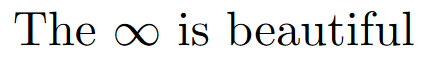How to write an infinity symbol in LaTeX?

# How to write an infinity symbol in LaTeX?

This post may contain affiliate links that allow us to earn a commission at no expense to you. Learn more

This article aims to show you a simple way to create an infinity symbol in LaTeX.

The infinity symbol can be seen most of the time in mathematics, like in limits or integrals involving boundaries. That’s why today you will learn how to insert the latex infinity symbol in LaTeX.

Just as many other symbols, the infinity symbol is built-in to LaTeX, that means you don’t need a package to write it in your document. The command is simple \infty, the math mode must be enabled (using the $to open and close it) and it does not take any value as argument. For example \begin{document} The$\infty$is beautiful \end{document}\infty output ## Infinity symbols in mathematics The infinity symbol can be used in many math topics, you will see some typical examples including, intervals, limits, integrals, and summations. For example \begin{document} Dom$\in \left(-\infty , 0 \right]\$ %interval with infinity
%---------------------------
$$\lim_{x\to\infty} = \frac{x^2+x-1}{x-8}$$ %limit with infinity
%---------------------------
$$\int_{-\infty}^{\infty}e^{-x^2}dx$$ %integral with infinity
%---------------------------
$$\sum_{n=0}^{\infty}\frac{(-1)^n}{(2n+1)!}\,x^{2n+1}$$ %summation with infinity, the sine series
\end{document}


As you can see it is straightforward and simple, maybe there could be other packages that include the symbol, but don’t complicate your LaTeX file if you don’t need to.

Now you have the knowledge of how to insert the infinity symbol or sign in LaTeX and as a plus some direct application of the infinity in mathematics.

I hope this post was helpful. If you have any questions leave them in the comments.

And, as always, keep writing in LaTeX